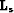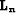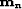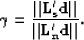Next: From filtering to covariance-pattern Up: Coherent noise filtering and Previous: Coherent noise filtering and

## The modeling approach

Before giving strategies on how to choose the modeling operatorsand, an important parameter needs to be introduced. Depending on the signal and noise that need to be attenuated, the two model spacesandcan have very different units. This difference can basically bias the inversion toward the noise or the signal if nothing is done to balance the two models. Therefore, a physically meaningful fitting goal for the modeling approach is(26)
whereis a constant that compensates for differences in the units ofand. This parameter can be seen as a way of putting the common components of the data in whichever domain we choose, noise or signal, in the presence of crosstalks. There is no acceptably simple way to selectbut by trial and error, e.g., in Chapter. One possible strategy, however, consists in computing the ratio of the norm (in asense) of the two gradients forandas follows:(27)
This ratio can be evaluated before starting the inversion Guitton (2000). Now for the choice of modeling operatorsand, it is important to have them modeling different parts of the data space. If they overlap, a regularization operator is needed to improve the signal/noise separation Nemeth (1996). There are a large number of operators that can model the signal and noise depending on the data. For instance, Nemeth et al. (2000) shows that migration can be used.

PEFs can play this role as well because they have the inverse spectrum of the data from which they were estimated. As an illustration, Figurea displays a simple monochromatic plane wave with a given dip. If a multidimensional PEF is estimated from this dataset, then the PEF will kill this plane wave (Figureb). Now, looking at the inverse impulse response of this filter (i.e., deconvolution) in Figurec, notice that an event with similar frequency content and dip than in Figurea is recovered. Thus, PEFs can serve as either filters when convolved with the residual (filtering approach) or modeling operators when used with deconvolution.

PEFs are minimum-phase filters Claerbout (1976); Robinson and Treitel (2000), which makes their inverse (with deconvolution) stable. In the stationary case where one filter is needed, inverse PEFs can play the role of modeling operators, and strategies similar to the one used for the filtering approach can be employed to estimate them. In the non-stationary case, however, the non-stationary deconvolution of minimum-phase filters is not guaranteed to be stable Rickett (2001). This defect prevents us from reliably using inverse non-stationary PEFs as modeling operators.

 fbpef Figure 1 Some basic properties of the prediction-error filters (PEF). (a) A PEF is estimated from a dataset. (b) The estimated PEF is convolved with the input data leading to a white residual. (c) A spike is deconvolved with the estimated PEF leading to a pattern close to the input data in (a).Next: From filtering to covariance-pattern Up: Coherent noise filtering and Previous: Coherent noise filtering and
Stanford Exploration Project
5/5/2005